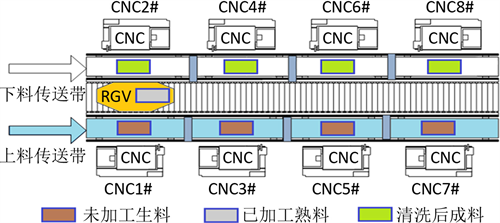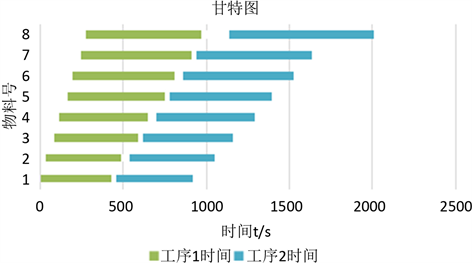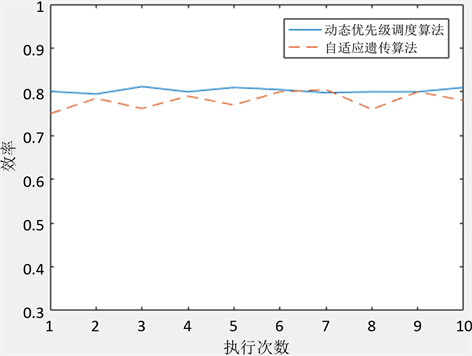﻿ 基于动态优先级算法的RGV调度策略

# 基于动态优先级算法的RGV调度策略Dynamic Scheduling Strategy Based on Dynamic Priority Algorithm

Abstract: The core of intelligent RGV dynamic scheduling strategy is to maximize the system efficiency, and priority determination of CNC machining is a powerful guarantee for maximizing efficiency. Based on the dynamic scheduling priority algorithm, this paper sets the priority selection criteria for the workbench by integrating the nearby principle, the shortest waiting time principle and the shortest feeding time principle. And taking the shortest task completion time as the goal, the paper gives a good answer to the path selection and the arrangement of the occurrence of fault in dynamic scheduling. Finally, Matlab is used to verify the maximum number of materials and the maximum working efficiency that can be processed within 8 hours under different cases of instance data. After verification, this strategy has good effectiveness.

1. 引言

2. RGV调度问题描述Figure 1. Schematic diagram of intelligent machining system

2.1. 工序过程

1) 智能加工系统通电启动后，RGV在CNC1#和CNC2#正中间的初始位置，所有CNC都处于空闲状态。

2) 在工作正常情况下，如果某CNC处于空闲状态，则向RGV发出上料需求信号；否则，CNC处于加工作业状态，在加工作业完成即刻向RGV发出需求信号。

3) RGV在收到某CNC的需求信号后，它会自行确定该CNC的上下料作业次序，并依次按顺序为其上下料作业，若此时完成作业，已加工出熟料，则进行清洗作业。

4) RGV在完成一项作业任务后，立即判别执行下一个作业指令。此时，如果没有接到其他的作业指令，则RGV就在原地等待直到下一个作业指令。

5) 系统周而复始地重复3)至4)，直到系统停止作业，RGV回到初始位置。

3. 约束条件

3.1. 变量描述

1) 决策变量x

$x=\left\{\begin{array}{l}1,机器正常工作\\ 0,机器故障\end{array}$ (1)

2) 决策变量y

$y=\left\{\begin{array}{l}1,机器故障\\ 0,机器正常工作\end{array}$ (2)

3.2. 约束模型的建立

$o.p$ $\mathrm{min}l$

$\text{s}\text{.t}\text{\hspace{0.17em}}\left\{\begin{array}{l}l=m+t+{t}_{c}+{t}_{r}\\ {t}_{b}=T-{t}_{u}-t\\ {t}_{r}=x\left({t}_{w}-{t}_{b}\right)+y\left(r+{r}_{t}-T\right)\\ T\ge {t}_{u}\\ {t}_{d}-{t}_{u}\ge m+t+{t}_{w}\\ {{t}^{\prime }}_{u}-{t}_{u}\ge {m}^{\prime }+t+{{t}^{\prime }}_{c}\end{array}$ (3)

4. 基于动态优先级算法的模型求解

4.1. 模型算法的建立

1.若CNC空闲

1-1若机器故障

a.物料数减1，不删除数据

1-2若CNC上无物料

a.更新上料开始时间，即总时间；

b.更新总时间：总时间+=上料开始时间；

c. CNC状态置位加工，有物料；

1-3若CNC有物料，则根据CNC物料工序道数：

a.更新总时间：总时间+=清洗时间；此时总时间即为下料开始时间；

b.更新此台CNC生产总时间：生产总时间+=需生产加工时间

c.若为最后一道工序，则生产物料总数加1

d.更新新物料上料开始时间，即总时间；

e.更新总时间：总时间+=需生产加工时间；

2.计算各CNC剩余加工时间

2-1若CNC故障

a.若总时间 < 故障结束时间

b.否则，剩余时间 = 0，CNC状态复位；

2-2若CNC空闲，则剩余时间为0；

2-3若CNC工作

a.若已加工时间 < 生产加工时间

b.否则，剩余时间 = 0，CNC状态复位；

3.动态调度，计算最优选择

3-1若CNC无物料

a.若CNC正常

b.若CNC故障

3-2若CNC有物料

4.求出最短调度时间及索引位置；

5. RGV到索引位置等待，更新时间，若此时总时间大于机床故障开始时间，则置位该机床的故障标志位，回到步骤1；

4.2. 模型算法的验证

$\text{s}\text{.t}\text{\hspace{0.17em}}\left\{\begin{array}{l}{d}_{0}-{u}_{0}\ge {m}_{i}+{y}_{n}+{t}_{ij}\\ {s}_{0}-{u}_{0}={y}_{0}+\left({u}_{1}-{s}_{0}\right)\\ {s}_{1}-{u}_{1}=\left({u}_{1}-{s}_{0}\right)+\left({s}_{1}-{s}_{0}\right)\end{array}$ (4)

5. 自适应遗传算法

6. 实例分析Table 3. Results of operational efficiency (unit time: seconds)Table 4. Optimal solution based on genetic algorithmFigure 2. Gantt diagram of genetic algorithmFigure 3. Efficiency comparison of the two algorithms

7. 模型的评价

7.1. 优点

1) 本文通过动态调度、分治算法的方式，使RGV在每执行完一个步骤之后，计算下一个最优步骤，可达到全局处于最优的状态，计算出最优调度模型，得到一个比较好的模型解。

2) 本模型考虑了多道工序的复杂情况，借鉴性强。

3) 本文所阐述的模型逻辑严谨，工序之间状况良好，具有较高的作业效率，且与实际关联密切，为实际生产提供了一个良好的可借鉴模型。

4) 本模型可用MATLAB软件加以验证，使我们的模型具有较高的可信度。

7.2. 缺点

1) 本模型参数与变量较多，算法较为繁琐。

2) 本模型算法偏向于暴力解决方式，对于大型工厂有较高的平台要求。

 龙锋. 基于自适应遗传算法的W公司仓库货位分配与优化研究[D]: [硕士学位论文]. 广州: 华南理工大学, 2015.

 聂峰, 程珩. 多功能穿梭车优化调度研究[J]. 物流技术, 2008, 27(10): 251-253.

 Gao, L., Zhang, G.H., Zhang, L.P., et al. (2011) An efficient Memetic Algorithm for Solving the Job Shop Scheduling Problem. Computers & Industrial Engineering, 60, 699-705.
https://doi.org/10.1016/j.cie.2011.01.003

 Xia, W. and Wu, Z. (2005) An Effective Hybrid Optimization Approach for Mul-ti-Objective Flexible Job-Shop Scheduling Problems. Computers & Industrial Engineering, 48, 409-425.
https://doi.org/10.1016/j.cie.2005.01.018

 Wu, L.H., Mok, P.Y. and Zhang, J. (2010) An Adaptive Multi-Parameter Based Dispatching Strategy for Single-Loop Interbay Material Handling Systems. Computers in Industry, 62, 175-186.
https://doi.org/10.1016/j.compind.2010.10.010

 陈华. 基于分区法的2-RGV 调度问题的模型和算法[J]. 工业工程与管理, 2014, 19(6): 70-77.

 林佳良. 基于现实的自动化立体仓库轨道式循环搬运系统构建与调度优化[D]: [硕士学位论文]. 北京: 北京物资学院, 2014.

 来学伟. 动态规划法在TSP问题中的应用[J]. 吉林化工学院学报, 2017, 34(3): 65-67.

 江唯, 何非, 童一飞, 李东波 基于混合算法的环形轨道RGV系统调度优化研究[J]. 计算机工程与应用, 2016, 52(22): 242-247.

Top